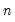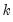impulse
Display impulse response functions of var object with an estimated VAR or VEC.
Syntax
var_name.impulse(n, options) ser1 [ser2 ser3 ...] [@ shock_series [@ ordering_series]]
You must specify the number of periodsover which to compute the impulse response functions.
List the series names in the var whose responses you would like to compute. You may optionally specify the sources of shocks. To specify the shocks, list the series after an “@”. By default, EViews computes the responses to all possible sources of shocks using the ordering in the Var.
If you are using impulses from the Cholesky factor, you may change the Cholesky ordering by listing the order of the series after a second “@”.
Options
General Options

 g Display combined graphs, with impulse responses of one variable to all shocks shown in one graph. m (default) Display multiple graphs, with impulse response to each shock shown in separate graphs. t Tabulate the impulse responses. a Accumulate the impulse responses. imp=arg (default=“chol”) Type of factorization for the decomposition: unit impulses, ignoring correlations among the residuals (“imp=unit”), non-orthogonal, ignoring correlations among the residuals (“imp=nonort”), Cholesky with d.f. correction (“imp=chol”), Cholesky without d.f. correction (“imp=mlechol”), Generalized (“imp=gen”), structural (“imp=struct”), or user specified (“imp=user”). The structural factorization is based on the estimated structural VAR. To use this option, you must first estimate the structural decomposition; see Var::svar.For user-specified impulses, you must specify the name of the vector/matrix containing the impulses using the “fname=” option.The option “imp=mlechol” is provided for backward compatibility with EViews 3.x and earlier. fname=name Specify name of vector/matrix containing the impulses. The vector/matrix must haverows and 1 orcolumns, whereis the number of endogenous variables. se=arg Standard error calculations: “se=a” (analytic), “se=mcarlo” (Monte Carlo), “se=boot” (bootstrap).If selecting Monte Carlo or bootstrap, you must specify the number of replications with the “rep=” option. Note the following: (1) Analytic standard errors are currently not available for (a) VECs and (b) structural decompositions identified by long-run restrictions. The “se=a” option will be ignored for these cases.(2) Monte Carlo standard errors are currently not available for (a) VECs and (b) structural decompositions. The “se=mcarlo” option will be ignored for these cases. (3) VECs only compute bootstrap standard errors so this option will be ignored. rep=integer Number of Monte Carlo or bootstrap replications to be used in computing the standard errors. Must be used with the “se=mcarlo” and “se=boot” options. bs=arg (default = “hp”) Bootstrap method: “sp” (standard percentile), “hp” (Hall’s percentile), “hs” (Hall’s studentized), “ku” (Killian’s unbiased). dbsrep (default = 499) Number of double bootstrap replications. Must be used with the “bs=hs” and “bs=ku” options unless the “fdb” option is specified, in which case this option will be ignored. fdb Approximate the double bootstrap computation using fast double bootstrap routines. cilevels=arg (default = “0.95”) Confidence interval coverage: space limited list of numbers between 0 and 1. uselines Use lines instead of shading for confidence intervals. matbys=name Save responses ordered by shocks (impulses) in a named matrix. The first column is the response of the first variable to the first shock, the second column is the response of the second variable to the first shock, and so on. The response and shock orderings correspond to the ordering of variables in the VAR. matbyr=name Save responses ordered by response series in a named matrix. The first column is the response of the first variable to the first shock, the second column is the response of the first variable to the second shock, and so on. The response and shock orderings correspond to the ordering of variables in the VAR. smat=name Save responses ordered by shocks (impulses) in a named matrix (akin to the “matbys=” option). The shocks and responses are ordered according to the user-specified order given by the “@ shock_series” and “@ ordering_series” specifications. rmat=name Save responses ordered by response series in a named matrix (akin to the “matbyr=” option). The shocks and responses are ordered according to the user-specified order given by the “@ shock_series” and “@ ordering_series” specifications. cimat=name Save matrix consisting of confidence intervals (lower-upper pairs for each impulse-response combination). rcimat=name Save matrix consisting of impulse responses and associated confidence intervals (lower-upper pairs for each impulse-response combination). prompt Force the dialog to appear from within a program. p Print the results.
BVAR Options

 bvartype = arg (default= “bayes”) Impulse method: Bayesian sampling (“bayes”), classical impulse response analysis using the posterior residual covariance matrix (“classpost”), classical impulse response analysis using the empirical residual covariance matrix (“classemp”).
If you are using Bayesian sampling, the following Bayesian options are available:

 draws=integer (default= 100000) Number of draws. burn=arg (default=0.1) Proportion of initial draws to discard. seed=integer Random number seed. dropunstable Drop any draws that produce unstable coefficients. dgraph Produce distribution graphs. page=arg Store the individual draws in a new page.
BTVCVAR Options

 starts = arg Set impulse dates. For multiple dates, enter a space-delimited list of values surrounded by quotation marks, e.g., "1980q1 2000q1 2020q1". usemean Use posterior mean as the point estimate. The posterior median is used if usemean is not included in the options list. showci Show credibility intervals (bands).
Examples
var var1.ls 1 4 m1 gdp cpi
var1.impulse(10,m) gdp @ m1 gdp cpi
The first line declares and estimates a VAR with three variables. The second line displays multiple graphs of the impulse responses of GDP to shocks to the three series in the VAR using the ordering as specified in VAR1.
var1.impulse(10,m) gdp @ m1 @ cpi gdp m1
displays the impulse response of GDP to a one standard deviation shock in M1 using a different ordering.
Cross-references
See “Vector Autoregression (VAR) Models” for a discussion of impulse responses in VARs.